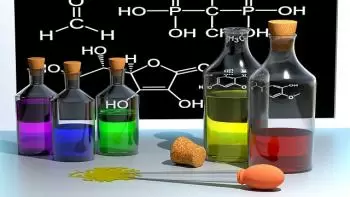# Ponderal Laws and Fundamental Laws of ChemistryThe fundamental laws of chemistry are those laws of nature relevant to chemistry.

The laws of stoichiometry, that is, the gravimetric proportions by which chemical elements participate in chemical reactions to form various compounds. Stoichiometric laws were proposed before Dalton's atomic theory and the concepts of the mole and molecular formula. Soon after, Dalton's theory would appear.

## Chemical Laws of Gases

Boyle's Law, which states that the pressure of a constant quantity of gas is inversely proportional to the volume.

Gay-Lussac's Law, which states that the variations in volume observed in a fixed quantity of a gas at constant pressure are directly proportional to the variations in temperature. This law is also known as the law of combining volumes.

## Ponderal Laws of Chemistry

Ponderal laws are classical laws of chemistry that refer to the quantitative aspects of the masses of different elements that combine to form other compounds in chemical reactions.

The ponderal laws are:

• Law of conservation of mass: Lavoisier's law.

• Law of definite proportions: Proust's law.

• Law of multiple proportions: Dalton's law.

• Reciprocal proportions law: Richter law.

Lavoisier's Law is also known as the law of conservation of mass. The law of conservation of matter states that the mass of a system does not change when it undergoes any transformation.

Law of constant proportions also defined as Proust's Law. This law establishes that in an isolated system the mass does not vary, that is, it remains constant. This property implies that the total mass of reactants is equal to the sum of the mass of the reaction products.

Dalton's law states that if two certain compounds form more than one compound with each other, then the masses of one of the elements corresponding to the same mass of the other element are related as simple integers.

Richter's law is also known as the law of equivalent proportions. This law establishes that the masses of the elements to be combined or substituted are proportional to their chemical equivalents.

Author:

Published: October 23, 2021
Last review: October 23, 2021